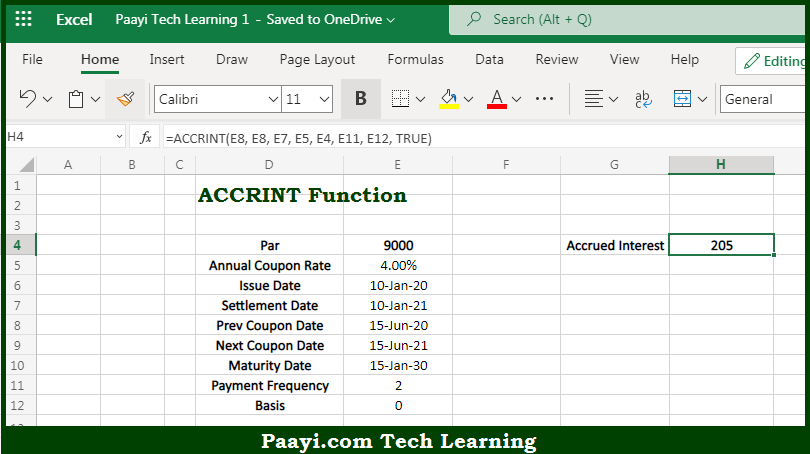# Learn How to Use Microsoft Excel ACCRINT Function

Written by | 0 Comments | 645 Views

In this article, you will learn how to use the Microsoft Excel ACCRINT function and its prime function in Microsoft Excel. You will also get to know the Microsoft Excel ACCRINT function return value and syntax with the help of some examples.

Microsoft Excel ACCRINT Function

The main purpose of the Microsoft Excel ACCRINT function is to get the accrued interest periodic. That implies, with the help of ACCRINT function you can able to return the accrued interest for a security that pays periodic interest. So, with the help of ACCRINT function, you can able to get accrued interest periodic.

Return Value of ACCRINT Function

The return value will be the accrued interest.

Syntax of ACCRINT Function

=ACCRINT(id, fd, sd, rate, par, freq, [basis], [cacl])

Where the arguments:

• id: This is the issue date of the security.
• fd: This is the first interest date of the security.
• sd: This is the settlement date of the security.
• rate: This is the interest rate of the security.
• par: This is the par value of the security.
• freq: This is the Coupon payments per year where, annual = 1, semiannual = 2, and quarterly = 4.
• basis: This is the day count basis, default is 0 (optional).
• calc: This is the calculation method (optional).

## How to Use Microsoft Excel ACCRINT Function?So we know that Microsoft Excel ACCRINT function you can able to get the accrued interest periodic. That implies, with the help of ACCRINT function you can able to return the accrued interest for a security that pays periodic interest. So, with the help of ACCRINT function, you can able to get accrued interest periodic.